## Saturday, January 05, 2008

### Roots of Unity: The Cyclotomic Polynomial

Since 1 is always a root of unity, we can determine the other roots of unity by dividing (xn - 1) by (x -1) and solving the equation.

In the case of n=2, this gives us: (x2 - 1)/(x - 1) = (x + 1). So, the other root of unity is x = -1.

In the case of n=3, this gives us: (x3 - 1)/(x - 1) = (x2 + x + 1). Using the quadratic equation to solve for this (see Theorem, here) gives us:

(-1 ± √1 - 4)/2 = (-1 ± √-3)/2.

In light of this and a previous result (see Lemma 1, here), it is possible to generate an equation to determine the primitive nth roots of unity.

Definition 1: Cyclotomic Polynomial Φn(x)

Φ1(x) = x - 1

Otherwise: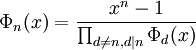where d includes all divisors of n except for n itself.

Lemma 1:

If p is prime, then:

Φp = xp-1 + xp-2 + ... + x + 1

Proof:

(1) Since p is prime, the only divisor is 1 and:

Φp = (xp - 1)/(x - 1)

(2) Now, we know that (xp - 1)/(x - 1) = xp-1 + xp-2 + ... + x + 1 since:

(a) Let s = xp-1 + xp-2 + ... + x + 1

(b) x*s = xp + xp-1 + ... + x2 + x

(c) x*s - s = xp - 1

(d) s(x - 1) = xp - 1

(e) s = (xp - 1)/(x - 1)

QED

Now, the next thing we need is a proof that Φn will generate a polynomial whose solution is the primitive n-th roots of unity:

Theorem 2: Cyclotomic Polynomial

For every integer n ≥ 1, Φn is a monic polynomial (see Definition 5, here for monic if needed) with integral coefficients and:

Φn = ∏(x - ζ) ∈ Z[x]

where ζ runs over the set of primitive n-th roots of unity and Z is the set of integers.

Proof:

(1) For Φ1=x-1, the theorem is clearly true.

(2) Let's assume that the theorem is true up to n-1.

(3) Since d is always less than n, for each d, we have:

Φd(x) = ∏(x - ζ) ∈ Z[x]

where ζ runs over the roots of unity with exponent d.

(4) Since each root of Φd is a root of unity, it follows that Φd divides xn - 1 [see Lemma 1, here]

(5) If d1, d2 are distinct divisors of n, then it follows that Φd1 and Φd2 are relatively prime polynomials and distinct -- that is, they do not include any common root of unity since:

Each root of unity has a unique exponent so no root of unity included in Φn will be found in more than one divisor Φdi.

(6) Using Corollary 2.2, here, it follows that:is a polynomial since it is divible by a product of all Φd

(7) Since each Φd is monic, it follows that Φn is monic.

(8) Since each Φd is in Z[x] and since xn - 1 is in Z[x], using the Division Algorithm for Polynomials, it follows that Φn is in Z[x]. [See Theorem, here]

(9) Now for each (x - ζi) that divides Φn, it follows that it's exponent equals n since:

(a) If its exponent is less than n and it is an n-th root of unity, then its exponent e is a divisor of n and it has been divided out.

(b) Assume that e doesn't divide n.

(c) So that n = qe + r where r ≥ 1 and r is less than e.

(d) Then:

(ζ)qe+r = 1 = (ζ)qe*(ζr)

(e) We know that (ζ)qe = 1 since:

(ζ)qe = (ζe)q

(f) But then:

ζr = 1/(ζqe) = 1

(g) This is impossible since r is less than e and e is the exponent of the root of unity.

(h) Therefore, we reject the assumption in 9b.

QED

References
• Jean-Pierre Tignol, Galois' Theory of Algebraic Equations, World Scientific, 2001

### Primitive Roots of Unity

In analyzing whether a root of unity is expressible as a radical, it is valuable to leverage the idea of a primitive root of unity. This root of unity has the following properties:

(1) The primitive n-th root of unity only equals 1 when it is raised to a power that is a multiple of n.
(2) If ζ is a primitive n-th root of unity, then the n distinct n-th roots of unity are: ζ0, ζ1, ..., ζn-1

Definition 1: Exponent e of an n-th root of unity

The exponent e of an n-th root of unity ζ is the smallest integer such that e is greater than 0 and ζe = 1.

Examples:

The exponent of 1 is 1.

The exponent of -1 is 2.

Definition 2: Primitive n-th roots of unity

An n-th root of unity is primitive if and only if its exponent is n.

Example:

-1 is a primitive square (second) root of unity since (-1)2 = 1.

1 is not a primitive square (second) root of unity since (1)1 = 1.

i, -i are primitive fourth roots of unity.

1, -1 are not primitive fourth roots of unity.

Lemma 1:

For a root of unity ζ, ζm = 1 if and only if its exponent e divides m.

Proof:

(1) Assume that e divides m.

(2) Then there exists f such that m = ef.

(3) So ζm = ζef = (ζe)f = (1)f = 1

(4) Assume that ζm = 1

(5) Let d = gcd(e,m)

(6) By Bezout's Identity (see Lemma 1, here), there exists r,s such that:

mr + es = d

(7) Now,

ζd = ζmres = (ζm)r*(ζe)s = (1)r*(1)s = 1

(8) But since e is the smallest number whereby (ζ)e = 1, it follows that e ≤ d.

(9) But since d divides e, it follows that d ≤ e.

(10) It therefore follows (see Lemma 2, here) that d = e.

(11) Since d divides m, it follows that e divides m.

QED

Theorem 2:

Let ζ, η be roots of unity of exponents e and f.

If e and f are relatively prime, then ζ*η is a root of unity of exponent e*f.

Proof:

(1) By definition 1 above:

ζe = 1

ηf = 1

so that:

(ζ*η)ef = (ζ)ef*(η)ef = (ζe)f*(ηf)e=(1)f*(1)e=1

(2) This shows that ζ*η is a root of unity.

(3) Let k be the exponent of (ζ*η).

(4) From Lemma 1 above, we know that k divides e*f.

(5) Since:

(ζ*η)k = 1

It follows that:

(ζ*η)k = ζkk = 1

So that:

ζk = η-k

(6) Raising both sides to the power of f gives us:

k)f = ζkf = (η-k)f = η(-kf) = (ηf)-k = (1)-k = 1

(7) So by Lemma 1 again, we see that e divides kf.

(8) But gcd(e,f)=-1 so e must divide k. [see Lemma 3, here]

(9) We can make the same argument in step #5 (by switching ζ and η) to show that f must also divide k.

(10) Since gcd(e,f)=1 and e divides k and f divides k, it follows (
see Lemma 1, here) that ef divides k.

(11) Since ef divides k and k divides ef, it follows (
see Lemma 2, here) that k=ef.

QED

Theorem 3: Powers of a Primitive N-th Root of Unity

Let ζ be a primitive n-th root of unity

Then:

every n-th root of unity is a power of ζ

Proof:

(1) Since ζ is an n-th root of unity, all powers of ζ are n-th roots of unity:

i)n = (ζ)in = (ζn)i = (1)i = 1

(2) Next, we will show that each of these n powers 0, ζ1, ..., ζn-1) are distinct.

(3) Assume that they are not distinct.

(4) Then, there exists a distinct i,j such that:

0 ≤ i,j ≤ n-1

and

i ≠ j

and

ζi = ζj

(5) Assume that j is greater than i.

(6) From step #4, we have:

1 = ζji = ζj-i

(7) But since j,i are less than n, it follows that j-i is greater than 0 and less than n.

(8) But this is impossible since by definition, we know that n is the exponent of ζ

(9) So, we reject our assumption in step #5

(10) We know that there are exactly n roots of unity by applying the Fundamental Theorem of Algebra which says that xn - 1 = 0 has exactly n roots. [See Theorem, here]

QED

References
• Jean-Pierre Tignol, Galois' Theory of Algebraic Equations, World Scientific, 2001

## Friday, January 04, 2008

### Roots of Unity: Expressed as Radicals

In a previous blog, I showed how the roots of unity can be stated as trigonometric functions. The question remains about when and under what conditions, they can be expressed as radicals. After all, this is clearly the case if n is a power of 2.

Abraham de Moivre made significant progress in investigating this question. In today's blog, I will show that we can answer the case of a composite number. If n is a nonprime such that n=rs and the r-th roots of unity can be expressed as radicals and the s-th roots of unity can be expressed as radicals, then it follows that the n-th root of unity can be expressed as radicals.

Lemma 1:

Let r,s be positive integers
Let μ1, μ2, ..., μr be the r-th roots of unity.
Let ζ1, ζ2, ..., ζs be the s-th roots of unity.

Then:

The rs-th roots of unity are of the form: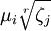for i = 1, ..., r and j = 1, ..., s.

Proof:

(1) Using an earlier result (see Lemma 1, here), we have: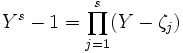(2) Setting Y = Xr gives us:(3) Using de Moivre's equation (see Theorem 1, here), we know that: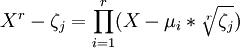(4) Combining step #2 and step #3, we have:QED

Theorem 2:

Let n be a positive integer.

If for each prime p factor of n, the p-th root of unity is expressible as radicals, then the n-th root of unity is expressible as radicals

Proof

(1) If n is a prime number, then the theorem is true by definition. So, for n=2, this theorem holds.

(2) Assume that there exists some number n such that the theorem holds true for all numbers less than n.

(3) If n is a prime, then the theorem is true and we are done. So, we assume that n is not a prime.

(4) Then there exists two numbers r,s such that r≠ 1 and s≠1 and n =rs.

(5) Since by assumption the r-th roots of unity and the s-th roots of unity are expressible by radicals, we can apply Lemma 1 above to conclude that n-th roots of unity must also be expressible by radicals.

QED

The implication of Theorem 2 is that to determine which n-th roots of unity are expressible as radicals, we only need to consider the cases where n is a prime number.

References
• Jean-Pierre Tignol, Galois' Theory of Algebraic Equations, World Scientific, 2001

## Tuesday, January 01, 2008

### Proof for Cotes' Formulas

In today's blog, I will show how De Moivre's Formula can be used to establish Cotes' Formulas.

Lemma 1:

Let μn be the set of all nth roots of unity

Then:Proof:

(1) We know that xn - 1 has n root from the Fundamental Theorem of Algebra.

(2) We also note that for all ζ, we have )n = 1

(3) Based on #2, the Fundamental Theorem of Algebra gives us:

xn - 1 = (x - ζ1)*(x - ζ2)*(x - ζ3)*...*(x - ζn)

(4) Step #3 can then be restated as:QED

Corollary 1.1:Proof:

This follows from applying De Moivre's proof [see Corollary 1.1, here] for the existence of the roots of complex numbers with the result in Lemma 1 above.

QED

Corollary 1.2: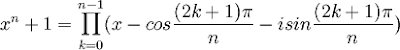Proof:

This follows from applying De Moivre's proof [see Corollary 1.2, here] for the existence of the roots of complex numbers with the result in Lemma 1 above.

QED

Theorem 2:

If n is odd: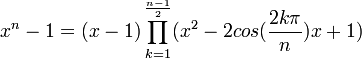If n is even: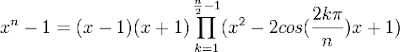Proof:

(1) From Corollary 1.1 above, we have:(2) Now, we can remove the k=0 case to get the following:(3) Now, it is also possible to pair up roots. That is, let l = n - k.

(4) Then we have:

cos(2[(n-k)π]/n) =
=cos([2nπ - 2kπ]/n) =
=cos([2nπ/n] - [2kπ/n]) =
=cos(2π - 2kπ/n) =
cos(-2kπ/n) = cos(2kπ/n) [See Property 9, here]

sin(2[(n-k)π]/n) =
=sin([2nπ - 2kπ]/n) =
=sin([2nπ/n] - [2kπ/n]) =
=sin(2π - 2kπ/n) =
=sin(-2kπ/n) = -sin(2kπ/n) [See Property 4, here]

(5) So, pairing the roots of unity k with its n-k pair gives us:(6) So, if n is odd, we have:(7) If n is even, then at k=n/2 we have:

cos([2(n/2)π]/n) = cos(nπ/n) = cos(π) = -1 [See Property 6, here]

sin([2(n/2)π]/n) = sin(nπ/n) = sin(π) = 0 [See Property 1, here]

So:

[x - cos([2(n/2)π];/n) - isin([2(n/2)π]/n)] =

= x - (-1) - i*0 = x + 1

(8) So, if n is even, we have:since we can see that n/2 is not included in any of the pairings:

k = 1 .. (n/2)-1

n-k = (n/2)+1 .. n-1

QED

Corollary 2.1:Proof:

(1) Assume that n is odd.

(2) Then from Theorem 2 above, we have:(3) Since n is odd, there exists m such that n = 2m+1.

(4) This then gives us:(5) Setting x = (a/x) gives us:(6) Multiplying both sides by x2m+1 gives us:(7) Assume that n is even.

(8) Then, from Theorem 2 above we have:(9) Since n is even, there exists an integer m such that n = 2m.

(10) This then gives us:(11) Setting x = (a/x) gives us: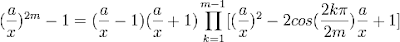(12) Multiplying both sides by x2m gives us:QED

Theorem 3:

If n is odd:If n is even: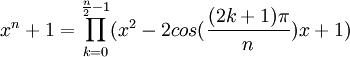Proof:

(1) From Corollary 1.2 above, we have:(2) Now, it is also possible to pair up roots. That is, let l = n - k -1.

(3) Then we have:

cos([2(n-k-1)+1]π/n) =
=cos([2n - 2k -1]π/n) =
=cos([2nπ/n] - [(2k+1)π/n] ) =
=cos(2π - (2k+1)π/n) =
cos(-[2k+1]π/n) = cos([2k+1]π/n) [See Property 9, here]

sin([2(n-k-1)+1]π/n) =
=sin([2n - 2k - 1]π/n) =
=sin([2nπ/n] - [(2k+1)π/n]) =
=sin(2π - (2k+1)π/n) =
=sin(-[2k+1]π/n) = -sin([2k+1]π/n) [See Property 4, here]

(4) So, pairing each roots of unity k with its n-k-1 pair gives us: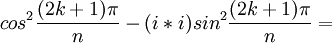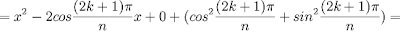(5) If n is even, the we have:(6) If n is odd, then at k=(n-1)/2:and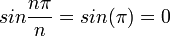(7) So:= x - (-1) - i*0 = x + 1

(8) So, if n is odd, we have:since we can see that (n-1)/2 is not included in any of the pairings:

k = 0 .. (n-1)/2 - 1

n-k-1 = (n-1)/2 + 1 .. n-1

since:

n-[(n-1)/2 - 1] - 1 =
= n - (n-1)/2 + 1 - 1 =
= (2n - [n-1])/2 =
= (n + 1)/2 =
= (n - 1 + 2)/2 =
(n-1)/2 + 1

QED

Corollary 3.1:Proof:

(1) Assume that n is odd.

(2) Then from Theorem 3 above, we have:(3) Since n is odd, there exists m such that n = 2m+1.

(4) This then gives us:(5) Setting x = (a/x) gives us: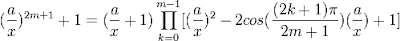(6) Multiplying both sides by x2m+1 gives us:(7) Assume that n is even.

(8) Then, from Theorem 3 above we have:(9) Since n is even, there exists an integer m such that n = 2m.

(10) This then gives us:(11) Setting x = (a/x) gives us:(12) Multiplying both sides by x2m gives us:QED

References
• Jean-Pierre Tignol, Galois' Theory of Algebraic Equations, World Scientific, 2001